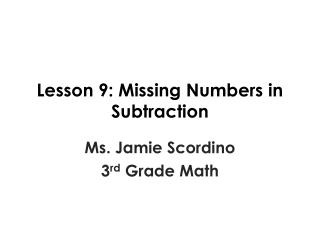DownloadDownload PresentationLesson 9: Missing Numbers in Subtraction

# Lesson 9: Missing Numbers in Subtraction

Download Presentation## Lesson 9: Missing Numbers in Subtraction

- - - - - - - - - - - - - - - - - - - - - - - - - - - E N D - - - - - - - - - - - - - - - - - - - - - - - - - - -
##### Presentation Transcript

1. Lesson 9: Missing Numbers in Subtraction Ms. Jamie Scordino 3rd Grade Math

2. Review: Missing Addends Keegan picked 14 apples. His friend, brought him some more. Now he has 34 apples. How many apples did his friend bring him?

3. Review: Missing Addends Keegan picked 14 apples. His friend, brought him some more. Now he has 34 apples. How many apples did his friend bring him? Number sentence: 14 + A = 34 -14- 14 0 + A = 20 A = 20

4. Solving Equations First way: Sometimes we just know the answer y - 6 = 8 We know y must be 14 to make this true. 14 – 6 = 8 This says some number minus 6 is equal to 8.

5. Solving Equations The opposite of subtraction is addition. We can add the number to both sides so we are left with our variable. Second way: y – 6 = 8 +6+6 y – 0 = 14 So y = 14

6. Problem #1 If we do something to one side, we MUST do the same thing to the other side. Need to add 5 to be left with the variable. r – 5 = 9 +5+5 r – 0 =14 r = 14

7. Your Turn h - 7 = 12

8. Solution Great Job! h - 7 = 1 2 + 7+7 h - 0 = 1 9 h = 19

9. Problem #2 (Tougher) If we do something to one side, we MUST do the same thing to the other side. Need to add 42 to be left with the variable. s - 42 = 41 + 42+42 s - 0 =83 s = 383

10. Your Turn z - 56 = 77

11. Solution Great Job! z – 56 = 7 7 + 56 +5 6 z - 0 = 133 z = 133

12. Word Problem #1 Kiran had some toy cars. He gave one car to each of his nine friends. Now he has 25 cars left. How many toy cars did Kiran have to start? Number Sentence: c-9 = 25 + 9+ 9 c - 0 = 34 c = 34

13. Word Problem #2 Mia had some crackers. She gave 15 to her best friend Aria. Now she has 29. How many crackers did Mia have to start? Number Sentence: c- 15 = 29 +15 +15 c - 0 = 44 c = 44

14. Your Turn Thank you for a great lesson!